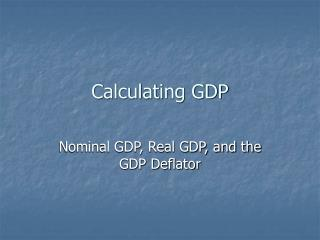DownloadDownload PresentationCalculating GDP

# Calculating GDP

Télécharger la présentation## Calculating GDP

- - - - - - - - - - - - - - - - - - - - - - - - - - - E N D - - - - - - - - - - - - - - - - - - - - - - - - - - -
##### Presentation Transcript

1. Calculating GDP Nominal GDP, Real GDP, and the GDP Deflator

2. There are two ways that GDP can increase: • An increase in the PRICES of goods and services. • An increase in the QUANTITY of goods and services. We need a method to calculate GDP that addresses rising prices

3. Our Simple Economy • Suppose an economy produces three goods or services, Window Washing, Baseballs, and Hammers. Data for the past three years can be found below.

4. Prices and Quantities for our Simple Economy

5. Nominal GDP Step 1: Calculate Nominal GDP (The value of final goods and services evaluated at current-year prices) for each year: NGDP2006 = Q2006 x P2006 = (90 x \$50.00) Window Washing + (75 x \$2.00) Baseballs + (50 x \$30.00) Hammers = \$6,150

6. Nominal GDP 2007 NGDP2007 = Q2007 x P2007 = (100 x \$60.00) Window Washing + (100 x \$2.00) Baseballs + (50 x \$25.00) Hammers = \$7,450

7. Nominal GDP 2008 NGDP2008 = Q2008 x P2008 = (100 x \$65.00) Window Washing + (120 x \$2.25) Baseballs + (65 x \$25.00) Hammers = \$8,395

8. Real GDP • Step 2: Calculate Real GDP (The value of final goods and services evaluated at base-year prices) for each year. For our example assume 2006 is the base year. This means that all values are in what we call “2006 Dollars”, or “Constant Dollars”.

9. Real GDP • By using the prices from the base-year, (or holding prices constant over time), we eliminate the impact that rising prices have on GDP, to get a measure of “Real” economic activity.

10. Real GDP in 2006 RGDP2006 = Q2006 x P2006 = (90 x \$50.00) Window Washing + (75 x \$2.00) Baseballs + (50 x \$30.00) Hammers = \$6,150 Note: For the Base-Year Nominal GDP always equals Real GDP

11. Real GDP in 2007 RGDP2007 = Q2007 x P2006 = (100 x \$50.00) Window Washing + (100 x \$2.00) Baseballs + (50 x \$30.00) Hammers = \$6,700 Note: We use “Current Quantities” and “Constant Prices”.

12. Real GDP in 2008 RGDP2008 = Q2008 x P2006 = (100 x \$50.00) Window Washing + (120 x \$2.00) Baseballs + (65 x \$30.00) Hammers = \$7,190 Note: We still use “Current Quantities” and “Constant Prices”.

13. The General Formula for Calculating a Growth Rate

14. Calculate the Growth Rate in Real GDP between 2006 and 2007 %Change = [(RGDP2007 – RGDP2006)/RGDP2006] x 100 %Change = [(6,700 – 6,150)/6,150] x 100 %Change = 8.94% That is real GDP grew by 8.94% between 2006 and 2007.

15. Calculate the Growth Rate in Real GDP between 2007 and 2008 %Change = [(RGDP2008 – RGDP2007)/RGDP2007] x 100 %Change = [(7,190 – 6,700)/6,700] x 100 %Change = 7.31% That is real GDP grew by 7.31% between 2007 and 2008.

16. The Price Level We can use our calculations of Nominal GDP and Real GDP to calculate the Price Level (A measure of the average prices of goods and services in the economy.)

17. The GDP Deflator One example of a measure of the average price level is the GDP deflator.

18. Calculate the GDP Deflator for 2006 GDP Deflator2006 = (NGDP2006/RGDP2006) x 100 GDP Deflator2006 = (6,150/6,150) x 100 = 100 Note: The GDP Deflator is always equal to 100 in the base-year. The Price Index is “unitless”

19. Calculate the GDP Deflator for 2007 and 2008 GDP Deflator2007 = (NGDP2007/RGDP2007) x 100 GDP Deflator2007 = (7,450/6,700) x 100 = 111.19 GDP Deflator2008 = (NGDP2008/RGDP2008) x 100 GDP Deflator2008 = (8,395/7,190) x 100 = 116.76

20. The Inflation Rate We can use the growth rate formula from previous to calculate the Inflation Rate (the Inflation Rate is The percentage increase in the price level from one year to the next.)

21. Calculate the Inflation Rate from 2006 to 2007 Inflation Rate Between 2006 and 2007 = [(GDP Def.2007 – GDP Def.2006)/GDP Def.2006] x 100 Inflation Rate Between 2006 and 2007 = [(111.19 – 100)/100] x 100 = 11.19 That is the inflation rate between 2006 and 2007 was 11.19%.

22. Calculate the Inflation Rate from 2007 to 2008 Inflation Rate Between 2007 and 2008 = [(GDP Def.2008 – GDP Def.2007)/GDP Def.2007] x 100 Inflation Rate Between 2007 and 2008 = [(116.76 – 111.19)/111.19] x 100 = 5.01 That is the inflation rate between 2007 and 2008 was 5.01%.

23. Nominal GDP in the U.S. 1947 to 2008

24. Real GDP in the U.S. 1929 to 2008